# Banach analytic space

An infinite-dimensional generalization of the concept of an analytic space, which arose in the context of the study of deformations of analytic structures (cf. Deformation). Here, the local model is a Banach analytic set, i.e. a subset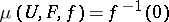of an open set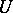in a Banach space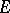over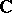, where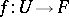is an analytic mapping into the Banach space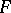. As distinct from the finite-dimensional case, not one structure sheaf, but a set of sheaves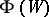, whereis an open set in an arbitrary Banach space, is defined on the local model.is defined as the quotient of the sheaf of germs of analytic mappings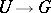by the subsheaf of germs of mappings of the type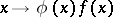, where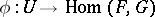is a local analytic mapping, while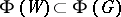is generated by mappings which assume values in. The sheavesdefine a functor from the categoryof open sets in Banach spaces and their analytic mappings into the category of sheaves of sets on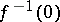.
A topological spacewith a functor from the categoryinto the category of sheaves of sets inin which all points have neighbourhoods isomorphic to some local model, is said to be a Banach analytic space.
Complex-analytic spaces form a complete subcategory in the category of Banach analytic spaces. A Banach analytic space is finite-dimensional if each one of its pointshas a neighbourhood that is isomorphic to a model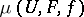and for which there exists a mappinginducing an automorphism of the model and having a completely-continuous differential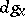.
A second special case of a Banach analytic space is a Banach analytic manifold, i.e. an analytic space that is locally isomorphic to open subsets of Banach spaces. An important example is the manifold of linear subspaces of a Banach space overthat are closed and admit closed complements.
Finitely-defined Banach analytic sets, i.e. models of the type, have local properties which correspond to classical properties: primary decomposition, Hilbert's Nullstellen theorem, the local description theorem, etc., are all applicable .